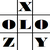anything in Excel...

It's Excel-o-lozy...

### Excel 2007: 1st of next month - How to calculate 1st day of next month from a specified date.

say Cell A1 contains "02 Feb 2012" as specified date..

Now a date is to be calculated which should be 1st day of month next to this date.

i.e. result should be "01 Mar 2012"…

How???
Easy…

use this formula "=EOMONTH(A1,0)+1"

EOMONTH is a formula for calculating "End Of MONTH" i.e. last day of 'Nth' month specified in number.

In the above formula, 0 (Zero) means same month and "+1" is the one day next to end of current month.

Super trick used simply... isn't it???

How to do it in Excel 2003, wait for next post.